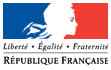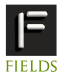# THEMATIC PROGRAMSJune  8, 2023## Thematic Program on O-minimal Structures and Real Analytic Geometry January 12 - 16, 2009 Winter School in O-minimal Geometry

Organized by Matthias Aschenbrenner

### Minicourse Abstracts

Supported by### Introduction to Model Theory Lecturer: Deirdre Haskell

The goal of these four lectures is to explain to the listener with no prior knowledge of mathematical logic enough of the key concepts of model theory to understand the definition and the power of the notion of o-minimality.

Lectures 1 and 2: language, structure, definable sets, elementary equivalence, model completeness and quantifier elimination, geometric interpretation, Hilbert's 17th problem, techniques for proving quantifier elimination

Lecture 3 and 4: Examples of proving model completeness/ quantifier elimination: real closed fields; the real field with restricted analytic functions; the real field with a predicate for integer powers of 2.

### INTRODUCTION TO REAL ANALYTIC GEOMETRY Lecturer: Krzystof Kurdyka

The goal of this course is to give a quick backround in real analytic geometry, more precisely to semi-analytic geometry which was created by S.Lojasiewicz in his celebrated IHES lecture notes  from 1965 (now available on web). Actually the lecture will be based on a more recent paper  of S. Lojasiewicz, M-A. Zurro and myself.

Lecture 1. Holomorphic and analytic functions in several variables. Power series, holomorphic functions, Riemann extension theorem, separate analyticity. References: , , .

Lecture 2. Preparation Theorem and Weierstrass polynomials. Continuity of roots, discriminant and generalized discriminant. Decomposition into irreducible factors. Puiseux’s theorem. References: , , , .

Lecture 3. Semi-analytic sets and distinguished stratifications. Real analytic sets, semi-analytic sets, Thom’s lemma, dimension. References: , , .

Lecture 4. Regular separation and Lojasiewicz’s inequality. Metric properties of seminalytic sets, curve selection lemma, Lojasiewicz’s inequality. References: , , .

References
 E. Bierstone and P. D. Milman, Semianalytic and Subanalytic sets, Publ. I.H.E.S.,
67 (1988), 5-42.
 M.Coste, Ensembles semi-alg´ebriques. Real algebraic geometry and quadratic forms
(Rennes, 1981), pp. 109–138, Lecture Notes in Math., 959, Springer, Berlin-New York,
1982
 R. Gunning, H. Rossi, Hugo Analytic functions of several complex variables. Prentice-
Hall, Inc., Englewood Cliffs, N.J. 1965 xiv+317 pp.
 K. Kurdyka, S. Lojasiewicz, M. Zurro Stratifications distingu´ees comme un util en
g´eom´etrie semi-analytique, Manuscripta Math. 86, 81–102, (1995).
 S. Lojasiewicz, Ensembles semi-analytiques,, preprint, I.H.E.S. (1965) available on
the web page ”http://perso.univ-rennes1.fr/michel.coste/Lojasiewicz.pdf”.
 S. Lojasiewicz, Introduction to complex analytic geometry, Birkh¨auser, Basel, 1991.
 R. Narasimhan, Introduction to the theory of analytic spaces. Lecture Notes in Math-
ematics, No. 25 Springer-Verlag, Berlin-New York 1966 iii+143 pp
 Ruiz, Jesus M. The basic theory of power series. Advanced Lectures in Mathematics.
Friedr. Vieweg & Sohn, Braunschweig, 1993. x+134 pp.

UNIVERSITE DE SAVOIE, Laboratoire de Mathematiques (LAMA), UMR
5127 CNRS, 73-376 Le Bourget-du-Lac cedex FRANCE

### Introduction to o-minimality. Lecturer: Sergei Starchenko

In these 4 lectures I intend to cover the following subjects.
I. Basics of o-minimality
(1) Monotonicity Theorem
(2) Cell decomposition
(3) Consequences of Cell decomposition.
(1) Triangulation and Euler Characteristic
(2) Piecewise smoothness
III. Hardy Fields, valuations and elementary extensions
(1.) Hardy fields, valuations and elementary extensions
(2.) Growth Dichotomy
(3.) Hausdorff Limits
(4.) Real closed valued fields
(5.) T-convexity
(6.) Bieri-Groves Theorem

### Analytic Vector Fields: Singularities and Limit Cycles Lecturer: Sergei Yakovenko

The minicourse is planned to provide the audience with the basic notions of real and complex analytic vector fields. We begin by describing different notions of normal forms for elementary singularities of vector fields and desingularization (blow-up) for non-elementary planar singularities. The minicourse will culminate with the proof of the finiteness theorem for limit cycles of polynomial vector fields having only nondegenerate singularities.

The material is covered in the recent textbook: Ilyashenko, Yakovenko, Lectures on Analytic Differential Equations (AMS 2007), Chapter I and Chapter IV (sect. 24).

The draft version of this textbook is available online at http://www.wisdom.weizmann.ac.il/~yakov/thebook1.pdf
Several first separate sections of the final version can be found on the blog http://yakovenko.wordpress.com/ (more specifically, at http://yakovenko.wordpress.com/category/lecture/ )